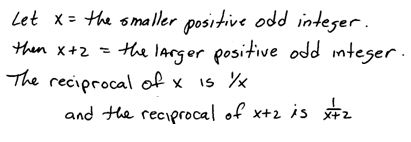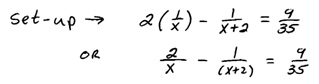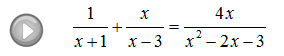## Algebra

Showing posts with label rational. Show all posts
Showing posts with label rational. Show all posts

### Polynomial and Rational Inequalities

Up to this point we have been solving quadratic inequalities.  The technique involving sign charts extends to solving polynomial inequalities of higher degree.

Solve.Step 1: Determine the critical numbers, which are the roots or zeros in the case of a polynomial inequality.
Step 2: Create a sign chart.
Step 3: Use the sign chart to answer the question.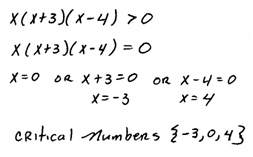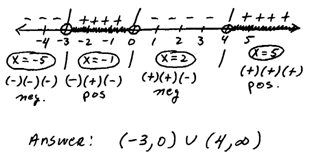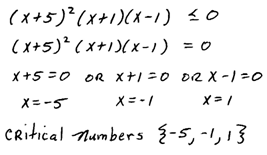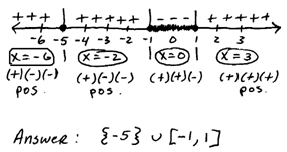The last problem shows that not all sign charts will alternate. Do not take any shortcuts and test each interval.

Rational inequalities are solved using the same technique.  The only difference is in the critical numbers.  It turns out that the y-values may change from positive to negative at a restriction. So we will include the zeros of the denominator in our list of critical numbers.

Solve.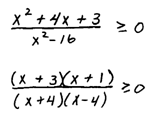Tip: Always use open dots for critical numbers that are also zeros of the denominator, or restrictions. This reminds us that they are restrictions and should not be included in the solution set even if the inequality is inclusive.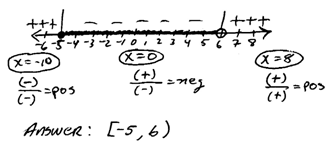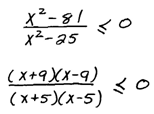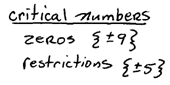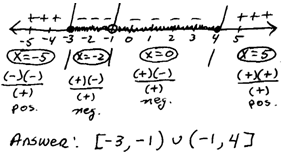Use open dots for all of the critical numbers when a strict inequality is involved.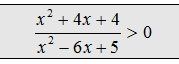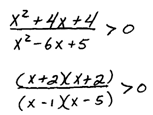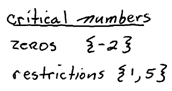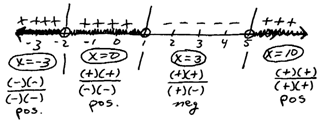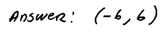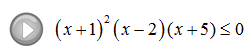### Solving Rational Equations

Rational equations are simply equations with rational expressions in them.

Use the technique outlined earlier to clear the fractions of a rational equation.  After clearing the fractions, we will be left with either a linear or a quadratic equation that can be solved as usual.

Step 1: Factor the denominators.
Step 2: Identify the restrictions.
Step 3: Multiply both sides of the equation by the LCD.
Step 4: Solve as usual.
Step 5: Check answers against the set of restrictions.This process sometimes produces answers that do not solve the original equation (extraneous solutions), so it is extremely important to check your answers.
Tip: It suffices to check that the answers are not restrictions to the domain of the original equation.

Solve.Determining the LCD is often the most difficult part of the process. Use one of each factor found in all denominators.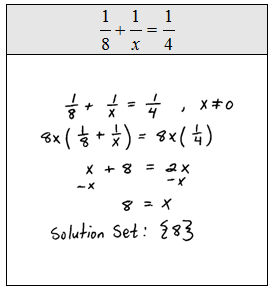Because of the distributive property, multiplying both sides of an equation by the LCD is equivalent to multiplying each term by that LCD as illustrated in the following examples.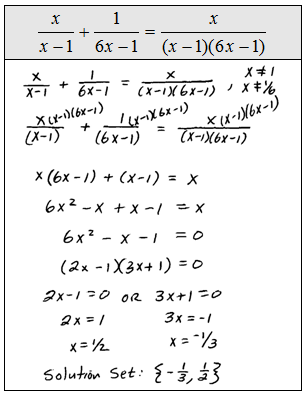Some literal equations, often referred to as formulas, are also rational equations. Use the techniques of this section and clear the fractions before solving for the particular variable.

Solve for the specified variable.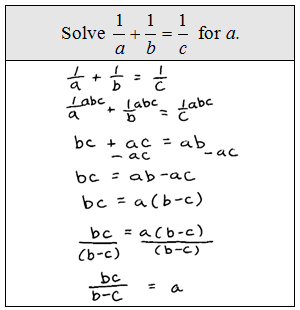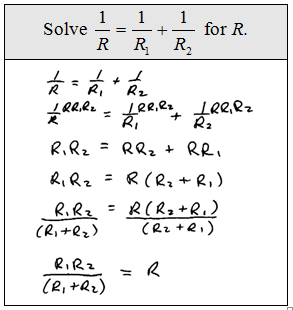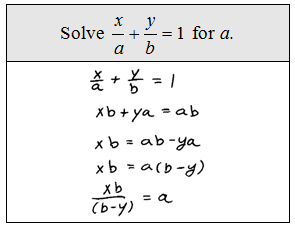The reciprocal of a number is the number we obtain by dividing 1 by that number.

Word Problem: The reciprocal of the larger of two consecutive positive odd integers is subtracted from twice the reciprocal of the smaller and the result is 9/35. Find the two integers.